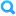This assignment does not count toward the final grade.

## RubricFind Rubric
Keep in mind that 7 students have already been assessed using this rubric. Changing it will affect their evaluations.
Rubric 2.1
Rubric 2.1
Criteria Ratings Pts
2.1 (a), (b), (c): Plot for (b) and log-log plot for (c), confirm O(n^3) for (c).
threshold: pts
 4.0 to >3.0 pts Both plots included and correct, correctly identified O(n^3) for part (c) with at least some reasoning included blank 3.0 to >2.0 pts Both plots included and correct, O(n^3) confirmed w/out at least a few words as to why. _4077 2.0 to >1.0 pts Both plots included, no confirmation of O(n^3) for (c). _798 1.0 to >0.0 pts One or both plots missing or incorrect and/or incorrect answer for (c). _8441 0.0 to >0 pts No plots or answer blank_2
pts
4.0 pts
--
2.1 (d) parts (a) and (b)
threshold: pts
 4.0 to >3.0 pts A + B identified as O(m*n) with reasoning as to the number of operations required to add the elements in two mxn matrices; Ax identified as O(n^2) with reasoning that counts the number of operations at each row. _1296 3.0 to >2.0 pts Correctly identified O(m*n) and O(n^2), with small logical errors in reasoning. _3777 2.0 to >1.0 pts Either correctly identified both O(m*n) and O(n^2) without any reasoning explained or incorrectly identified one of them, but with some explanation provided for both. _4493 1.0 to >0.0 pts Incorrectly identified both but an effort was made to explain the thought process _3627 0.0 to >0 pts No answer _1020
pts
4.0 pts
--
Total Points: 8.0 out of 8.0
 I'll write free-form comments when assessing students Remove points from rubric Don't post Outcomes results to Learning Mastery Gradebook Use this rubric for assignment grading Hide score total for assessment results
 I'll write free-form comments when assessing students Remove points from rubric Don't post Outcomes results to Learning Mastery Gradebook Use this rubric for assignment grading Hide score total for assessment results